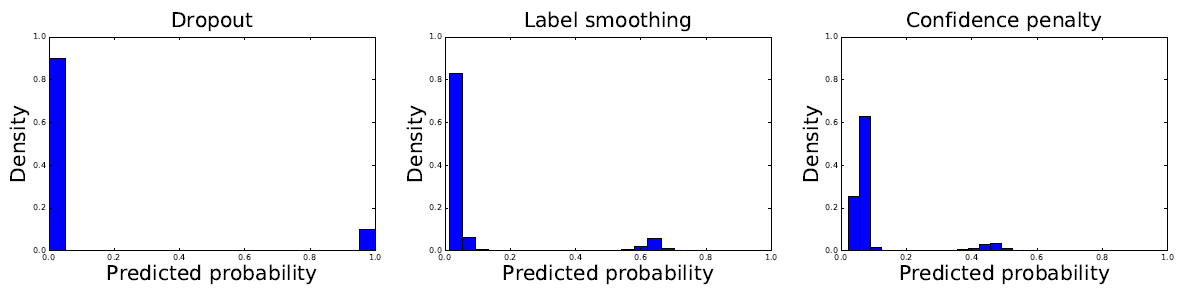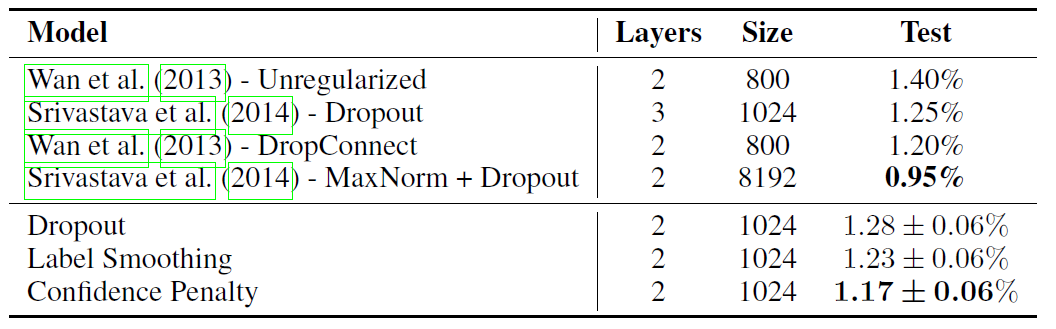This post is a summary and paper skimming on regularization and optimization. So, this post will be keep updating by the time.

# Regularizing neural networks by penalizing confident output distributions

• Conference: ICLR2017

## Summary

• Research Objective
• To suggest the wide applicable regularizers
• Proposed Solution
• Regularizing neural networks by penalizing low entropy output distributions
• Penalizing low entropy output distributions acts as a strong regularizer in supervised learning.
• Connect a maximum entropy based confidence penalty to label smoothing through the direction of the KL divergence.
• When the prior label distribution is uniform, label smoothing is equivalent to adding the KL divergence between the uniform distribution $u$ and the network’s predicted distribution $p_\theta$ to the negative log-likelihood.
• By reversing the direction of the KL divergence in equation (1), $D_{KL}(u \parallel p_\theta(y \mid x))$, it recovers the confidence penalty.Figure: Distribution of the magnitude of softmax probabilities on the MNIST validation set. A fully-connected, 2-layer, 1024-unit neural network was trained with dropout (left), label smoothing (center), and the confidence penalty (right). Dropout leads to a softmax distribution where probabilities are either 0 or 1. By contrast, both label smoothing and the confidence penalty lead to smoother output distributions, which results in better generalization.

• Contribution
• Both label smoothing and the confidence penalty improve state-of-the-art models across benchmarks without modifying existing hyperparametersFigure: Test error (%) for permutation-invariant MNIST.